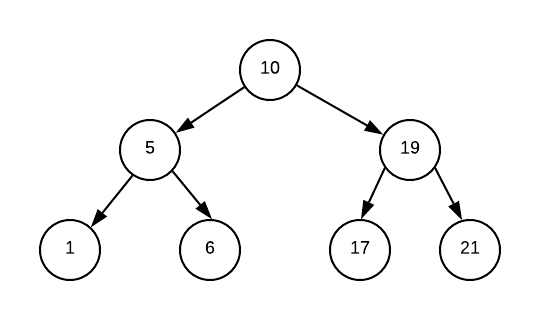# 二叉树先序中序后序的非递归实现Kenn

﻿

﻿

# 先序和中序遍历

  func handle(v int) {      fmt.Println(v)  }  func tranverse(root *TreeNode) []int {	cur := root	s := newStack()	for cur != nil || !s.isEmpty() {            for cur != nil {                s.push(cur)                //先序                //handle(cur.Val)                cur = cur.Left            }            cur = s.pop()            //中序            //handle(cur.Val)            cur = cur.Right	}    return arr}

﻿﻿

1.  //push 10

2. [10, 5] //push 5

3. [10, 5, 1] //push完1之后，cur == nil,会跳出循环

4. [10, 5] //pop出1, 接下来cur设为1.right，也就是nil

5.  //此时cur == nil, 会跳出循环, pop出5, cur为5.right, 也就是6

6. [10, 6] //push6之后， cur == nil, 会跳出循环

7.  //pop出6，接下来cur设为6.right，也就是nil

8. [] //pop出10，接下来cur设为10.right, 也就是19

9.  //push 19

10. [19, 17] //push 17之后，cur == nil，然后重复上面的步骤直到stack为空

﻿

﻿

# 统一的形式

﻿

func traversal(root *TreeNode) []int {    cur := root    s := newStack()    pre := cur    for cur != nil || !s.isEmpty() {        for cur != nil {            //先序遍历            //handle(cur.Val)            s.push(cur)            cur = cur.Left        }                                       cur  = s.seek()          //中序遍历        //if cur.Right != pre {            //  handle(cur.Val)        //}        cur = cur.Right         if cur == nil || cur == pre {            pre = s.pop()            //后序遍历            //handle(pre.Val)            cur = nil        }    } return arr}

﻿

		if cur == nil || cur == pre {			pre = s.pop()   			//后序遍历			//handle(pre.Val)			cur = nil		}

﻿

﻿

﻿

1. [10, 5, 1]

   cur  = s.seek() //此时cur为1      //中序遍历    //if cur.Right != pre {        //  handle(cur.Val)    //}    cur = cur.Right  //此时cur为nil    if cur == nil || cur == pre {        pre = s.pop()  //pre为 1         //后序遍历        //handle(pre.Val)        cur = nil    }
1. [10, 5]

   cur  = s.seek() //此时cur为5, 第一次访问父节点    //中序遍历    //if cur.Right != pre {        //  handle(cur.Val)    //}    cur = cur.Right  //此时cur为6, pre为1    //接下来会把cur push到stack里面去并跳出循环
1. [10, 5, 6]

   cur  = s.seek() //此时cur为6    //中序遍历    //if cur.Right != pre {        //  handle(cur.Val)    //}    cur = cur.Right  //此时cur为nil    if cur == nil || cur == pre {        pre = s.pop()  //pre为 6         //后序遍历        //handle(pre.Val)        cur = nil    }
1. [10, 5]

   cur  = s.seek() //此时cur为5, 第二次访问父节点    //中序遍历    //if cur.Right != pre {        //  handle(cur.Val)    //}    cur = cur.Right  //此时cur为6, pre 也为6    //此时cur == pre, 代表右节点已经访问过了, 我们需要pop    if cur == nil || cur == pre {        pre = s.pop()  //pre为 5         //后序遍历        //handle(pre.Val)        cur = nil    }

﻿

# 总结

﻿Démon de Laplace

## 评论